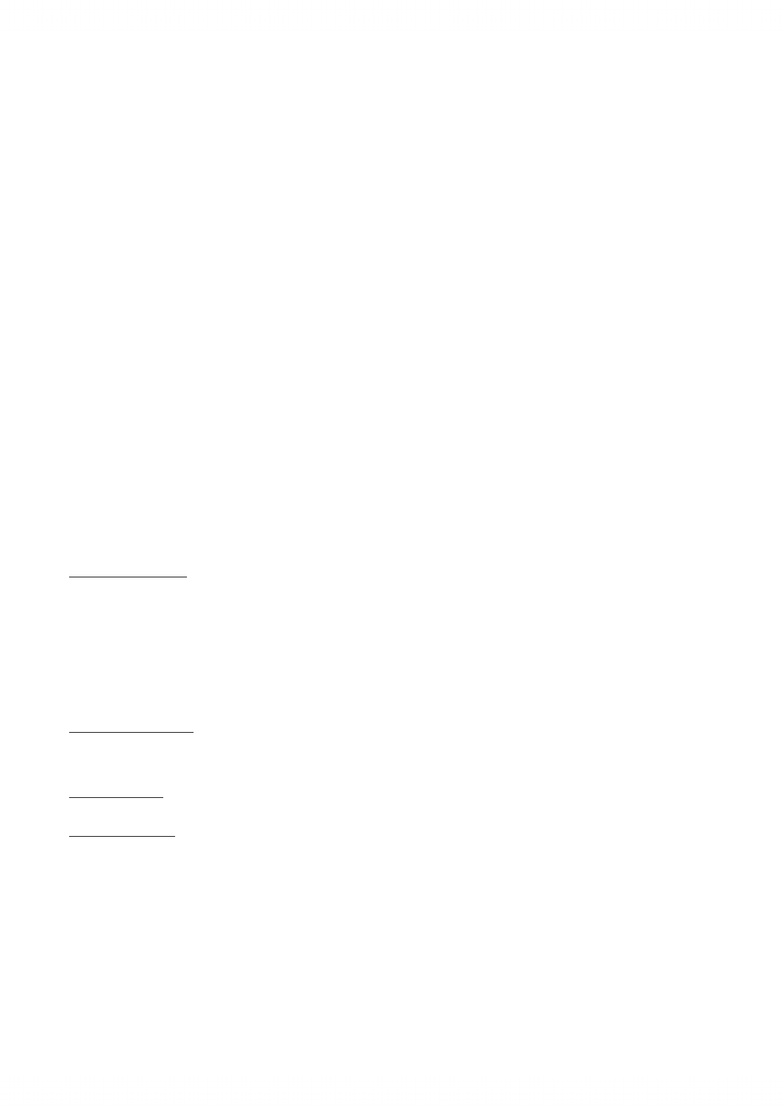Textbook Notes (290,000)
CA (170,000)
York (10,000)
ECON (800)
ECON 1000 (400)
Chapter 4

# Chapter 4 - ECON 1000

Department
Economics
Course Code
ECON 1000
Professor
Sam Lanfranco
Chapter
4

This preview shows page 1. to view the full 4 pages of the document.Price Elasticity of Demand
ELASTICITY = HOW MUCH DEMAND FOR AN ITEM CHANGES WHEN PRICE CHANGES
Does price fall by a large amount and quantity increase by a little?
Does price barely fall and quantity increase by a large amount?
When supply increases equilibrium price falls and equilibrium quantity increases
Different outcomes arise from differing degrees of responsiveness of the quantity demanded to a change in price
Can compare slopes of two demand curves
If supply increases the same in both cases
Soft slope = small fall in price and a large increase in quantity
A demand curve with a very steep slope V. demand curve with a softer slope
Example: slope
Slope depends on units in which we measure for price and quantity
Often need to compare demand for different goods and services that are measured in unrelated units
Example: pizza producer wants to compare demand for pizza with demand for soft drinks
Cannot always use slope
Use elasticity as a measure
Answer depends on responsiveness of the quantity demanded to a change in price
Units-free measure of the responsiveness of the quantity demanded of a good to a change in its price when all other influences on buying plans remain the
same
Price elasticity of demand = percentage change in quantity demanded / percentage change in price
Calculated using the formula
Price falls to \$19.50 a pizza and quantity demanded increases to 11 pizzas an hours
By using this you can calculate elasticity at a point on the demand curve midway between original and new point
To calculate we express changes in price and quantity demanded as percentages of average price and average quantity
Average of 20.50 and 19.50 = 19.5 \$20
\$1 price decrease is 5 percent of average price
\$1/\$20 = \$0.05 5%
Average of 9 and 11 = 11 10
2 increase in quantity demanded is 20 percent of average quantity
2/10 = 0.2 20%
Math:
Price elasticity of demand = 20%/5% = 400% = 400% = 4
When price falls by \$1 a pizza the quantity demanded icnreases by 2 pizzas an hour
Example: pizza is initially sold at \$20.50 and 9 sold in an hour
Price elasticity of demand:
Average Price and Quantity
At the midpoint between original and new price
Using average provides most precise measurement of elasticity
\$1 price change is 4.9% of \$20.50
This means elasticity of 22.2%/4.9%=453.0612% = 4.5
2 pizza change in quantity is 22.2% of 9 pizzas
Example: if price falls from \$20.50 to \$19.50
The \$1 price change is 5.1% of \$19.50
This means elasticity of 18.2%/5.1%=356.8627% = 3.6
The 2 pizza change in quantity is 18.2 percent of 11 pizzas
Example: if the price rises from \$19.50 to \$20.50
By using average you get same value of elasticity regardless if price falls from \$20.50 to \$19.50 or rises from \$19.50 to \$20.50
Percentages and Proportions
Elasticity is the ratio of two percentage changes
A percentage change is a proportionate change multiplied by 100
Divide the two proportions and you get same answer as using percentage change
Proportionate change in price is deltaP/Paverage and proportionate change in quantity demanded is delta Q/Q average
A Units-Free Measure
It is units-free because the percentage change is independent of units in which variable is measured
The ratio of the two percentages is a number without units
Minus Sign and Elasticity
When price of a good rises the quantity demanded decreases
Price elasticity is a negative number
So magnitude of elasticity is used and minus sign is ignored
The magnitude/absolute value of elasticity tells us how responsive the quantity demanded is
Positive change in price brings negative change in quantity demanded
Inelastic and Elastic Demand
Three demand curves cover entire range of possible elasticities of demand
If price changes quantity demanded remains the same
Price elasticity of demand is zero and it has perfectly inelastic demand
Example: insulin
Vertical Line
Price elasticity is 1 and it has unit elastic demand
If percentage change in quantity demanded equals percentage change in price
Elasticity of demand is between 1 and infinity and has elastic demand
Example: automobile and furniture
When percentage change in quantity demanded is more than the percentage change in price
Curved downwards
Horizontal Line
Chapter 4 - Elasticity
October-11-11
4:19 PM
ECON 1000 Page 1